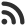Feed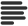Articles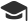Tutorials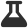Lab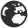CompaniesLeaderboard
 VK Programmer and Softwere Engineer at None Updated Jan. 1, 2021, 6:25 p.m. ⋅ 115 views

# TCS NQT/Digital Coding Program- 1 The product of Quadruplet

*A non-empty arrary A consisting of numeric values is given.

The product of quadruplet (P,Q,R,S) equates to A[P] * A[Q] * A[R] * A[S]

(0 <= P < Q < R < S < N).

For Example, Arrary A Such that.

A = -3, A  = 1,  A = 2,  A = -2, A = 5, A = 6, A = 1

• (0, 1, 2, 3) , product is  -3 * 1 * 2 * -2 = 12
• (1, 2, 4, 5) , product is   1 * 2 * 5 * 6 = 60
• (2, 4, 5, 6) , product is   2 * 5 * 6 * 1 = -60

60 is the product of quadruplet (2, 4, 5, 6), which is maximal.

Your goal is to find the maximal product of any quadruplet for input ArraryA[].

write an efficient algorithm for the following assumptions.

• N is an integer/float within the range[4 ..... 100,000];

Solution Code:-

number = int (input ("Enter count of value you are going to insert:"))

print ("Enter", number, "Numbers :-")

elements = [int (input()) for i in range (0, number)]

print (elements)

val = []

if (number >= 4):

for indexA in range (0, number):

listA = []

listB = []

if (number-indexA >= 4):

print ("indexA =", indexA)

listA.append (elements [indexA])

listB.append (indexA)

for indexB in range (indexA + 1, number):

if elements [indexA] <= elements [indexB]:

listA.append (elements [indexB])

listB.append (indexB)

print ("break")

break

if (len (listB) == 4):

print (listB , listB , listB, listB ,"......", listA,"*", listA, "*", listA, "*", listA, "-", listA * listA * listA *listA)

val.append (listA * listA * listA *listA)

listA.clear ()

listB.clear ()

print (" Maximum: ", max(val))

else:

print (" Invalid Input ")

Events

Nov. 28, 2018, 5:30 p.m.

Python from zero to hero

place Delhi

Aug. 13, 2018, 5:30 p.m.

Python from zero to hero

place Bangalore ( HackersFriend office BTM Layout)